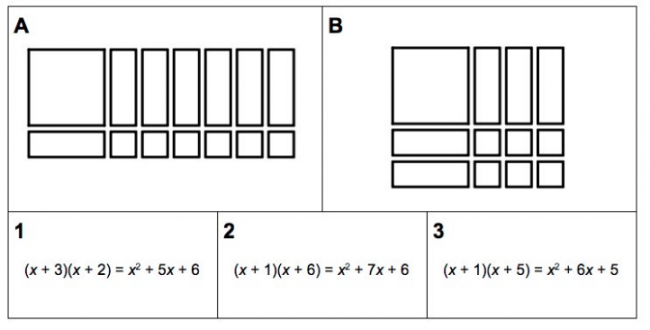# Area Model and Factoring #2

Preview:This task helps students build an area model of quadratic expressions for both unfactored and factored forms of a quadratic expression. This version includes negative coefficients in the factoring and uses color to distinguish between positive and negative terms.

One potential modification for this task is to remove the unsimplified in-between step between the factored and unfactored forms and let students determine what those forms should be during the exploration phase.

Connecting Representations
Content:
Curricular Competency:
Reasoning and analyzing: Develop mental math strategies and abilities to make sense of quantities
Understanding and solving: Develop, demonstrate, and apply mathematical understanding through play, inquiry, and problem solving
Understanding and solving: Visualize to explore mathematical concepts
Understanding and solving: Develop and use multiple strategies to engage in problem solving
Communicating and representing: Communicate mathematical thinking in many ways
Communicating and representing: Use mathematical vocabulary and language to contribute to mathematical discussions
Communicating and representing: Explain and justify mathematical ideas and decisions
Communicating and representing: Represent mathematical ideas in concrete, pictorial, and symbolic forms
Connecting and reflecting: Reflect on mathematical thinking